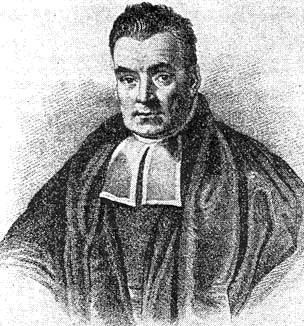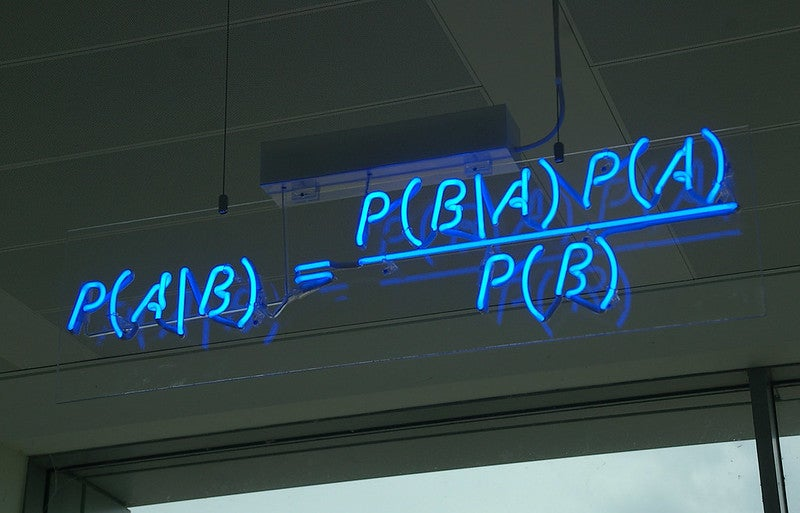# Introduction

What is this course? It is a crash course in brms: a brief and practical introduction to fitting Bayesian multilevel models in R and Stan using the brms package (Bayesian Regression Models using Stan). Because this is intended as a practical skills course, I will only give a quick and surface-level description of Bayesian analysis and how it can be used for multilevel regression models.

## What should you know coming into this course?

This course is designed for practicing researchers who have some experience with statistical analysis and coding in R.

### Minimal prerequisites

• You should have at least heard of a mixed model or multilevel model.
• You should have at least tried to do stats and/or data science in R before.
• You should have at least a vague knowledge of what Bayesian analysis and inference are.

• If you have experience with the R package lme4, that’s great because the syntax of brms is modeled after lme4.
• If you have experience with the tidyverse packages, including ggplot2, for manipulating and plotting data, that’s also great because we will be using them in this course.

If you don’t know what those packages are, don’t sweat it because you can just follow along by copying the code.

## What will you learn from this course?

### Conceptual learning objectives

At the end of this course, you will understand …

• The very basics of Bayesian inference
• What a prior, likelihood, and posterior are in the context of a Bayesian model
• The very basics of how Markov Chain Monte Carlo works
• What a credible interval is

### Practical skills

At the end of this course, you will be able to …

• Write the code to specify and sample a multilevel model with random intercepts and random slopes in brms
• Generate plots and statistics to make sure your model converged, and know a few ways to deal with convergence problems
• Interpret the summary output of a multilevel model in brms
• Compare different models with leave-one-out information criteria
• Use Bayes factors to assess strength of evidence for effects
• Make plots of model parameters and predictions, with credible intervals to show uncertainty

## What is Bayesian inference?This may or may not actually be a portrait of Thomas Bayes

In its simplest form, Bayesian inference is a method of statistical inference that allows you to use information you already know to assign a prior probability to a hypothesis, then update the probability of that hypothesis as you get more information. If that seems like common sense, it is!

It is a very powerful tool that has been applied across many fields of human endeavor. Today we are only going to look at its application in estimating the parameters of multilevel statistical models to analyze scientific data, but that’s only one of the many things we can use Bayesian inference for. Bayesian methods are only growing in popularity, thanks in large part to the rapid growth of user-friendly, open-source, computationally powerful software – like Stan and its companion R package brms that we are going to learn about today!

Bayesian inference is named after Reverend Thomas Bayes, an English clergyman, philosopher, and yes, statistician, who wrote a scholarly work on probability (published after his death in 1763). His essay included an algorithm to use evidence to find the distribution of the probability parameter of a binomial distribution … using what we now call Bayes’ Theorem! However, much of the foundations of Bayesian inference were really developed by Pierre-Simon Laplace independently of Bayes and at roughly the same time. Give credit where it’s due!

## Bayes’ TheoremBayes’ Theorem is the basis of Bayesian inference. It is a rule that describes how likely an event is to happen (its probability), based on our prior knowledge about conditions related to that event. In other words, it describes the conditional probability of an event A occurring, conditioned on the probability of another event B occurring.

The mathematical statement of Bayes’ theorem is:

$P(A|B) = \frac{P(B|A)P(A)}{P(B)}$

This means the probability of A being true given that B is true ($$P(A|B)$$) is equal to the probability that B is true given that A is true ($$P(B|A)$$) times the ratio of probabilities that A and B are true ($$\frac{P(A)}{P(B)}$$).

This may not make sense yet but some more concrete examples might help.

## How Bayes’ theorem relates to statistical inference

Let’s say $$A$$ is a statistical model. A statistical model is a hypothesis about the world. We want to know the probability that our hypothesis is true. We start out with some prior knowledge about the probability that our model $$A$$ is true. So $$P(A)$$ is called the prior probability. We go out there and get some data $$B$$ to test our hypothesis. We can calculate the probability of observing that data $$B$$, given that $$A$$ is true: $$P(B|A)$$. This is also known as the likelihood of the model $$A$$, in light of our observations $$B$$. We use this to update our estimate of the probability that $$A$$ is true. We now have $$P(A|B)$$, the probability our model, or hypothesis, is true, given the data we just observed.

Notice we ignored the denominator, $$P(B)$$, the probability that the data are true. This is called the marginal probability. We can usually ignore it in the context of statistical inference. That’s because the best we can do is to compare the relative probability of different models, given the data. We can’t really say (nor should we say) what the absolute probability of a model is. (“All models are wrong.”) If we are comparing two models $$A_1$$ and $$A_2$$, both fit using the same data $$B$$, and we take the ratio $$\frac{P(A_1|B)}{P(A_2|B)} = \frac{P(B|A_1)P(A_1)/P(B)}{P(B|A_2)P(A_2)/P(B)}$$, the two $$P(B)$$s cancel out. Thus we don’t really need to care about $$P(B)$$ in practice.

So we can get rid of the denominator and write Bayes’ theorem as

$P(model|data) \propto P(data|model)P(model)$

or

$posterior = likelihood \times prior$

or, flipping the left and right sides around,

what we believed before about the world (prior) × how much our new data changes our beliefs (likelihood) = what we believe now about the world (posterior)

That’s pretty cool!

## A “real-life” example of Bayes’ theorem

Let’s say we pick up a coin off the street. We have a very strong prior belief that the true probability of flipping heads on any given flip is 0.5. Let’s say we flipped the coin 10 times and got 8 heads. We probably wouldn’t change that belief very much based on that evidence. It simply isn’t enough evidence to sway us off that belief. The likelihood of getting 8 heads, given that the true probability is 0.5, is still high enough that our posterior estimate of the probability is still around 0.5.

But let’s say a shady character on the street corner approaches us, shows us a coin, and offers to flip the coin 10 times. He offers to pay us $1 for every tails if we pay him$1 for every heads. Most likely, before we even got any new evidence, we’d be likely to think that the true probability of heads is not exactly 0.5. Or at least we would have a more skeptical prior belief, and we would be willing to entertain the possibility that the true probability of heads might be higher than 0.5. So now if we observe 8 heads, we might update our estimate of the true probability of heads to something higher, maybe 0.7.